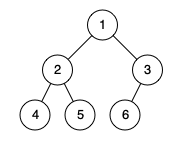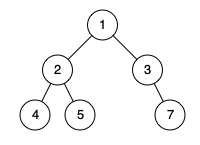# LeetCode: 958. 二叉树的完全性检验¶

## 1、题目描述¶输入：[1,2,3,4,5,6]输入：[1,2,3,4,5,null,7]



• 树中将会有 1 到 100 个结点。

## 2、解题思路¶

• 使用层次遍历
• 给节点编号，非最后一排都需要满节点
• 最后一排不能有空节点
# Definition for a binary tree node.
# class TreeNode:
#     def __init__(self, x):
#         self.val = x
#         self.left = None
#         self.right = None

class Solution:
def isCompleteTree(self, root: TreeNode) -> bool:
temp = [(root, 1)]
level = 0
while temp:

t = []
for node, index in temp:
if node.left:
t.append((node.left, 2 * index))
if node.right:
t.append((node.right, 2 * index + 1))

# 左侧验证
if temp != 2 ** level:
return False
# 验证没有空节点
if (temp[-1] - temp + 1) != len(temp):
return False
# 不是最后一排需要满节点
if t and (temp[-1] - temp + 1) != 2 ** level:
return False

level += 1
temp = t

return True